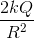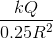MCAT Physical : Electrostatics and Electrical Fields

Example Questions

← Previous 1 3

Example Question #1 : Electrostatics And Electrical Fields

Two charges of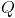coulombs are a distance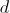apart from each other. Which of the following would reduce the force exerted between the charges by a factor of 4?

Increase distance d by a factor of 4.

Decrease distance D by a factor of two.

Decrease the each charge Q by a factor of four.

Increase distance D by a factor of two.

Increase distance D by a factor of two.

Explanation:

Given Coulomb's Law electrostatic forces: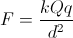We can see that distance and force are inversly related. Also distance is squared, so if we increase the distance by 2, the force between the two charges will be reduced by a factor of four.

Example Question #2 : Electrostatics And Electrical Fields

Two charges Q1 and Q2 are held stationary 1 m away from each other. The magnitude of charge of Q2 is twice that of Q1. A positive point charge A is placed directly in the middle of the two charges (Q1 and Q2). What is the ratio of the net force on charge A when Q1 and Q2 are both negative over the net force on charge A when Q1 is negative and Q2 is positive?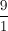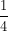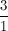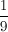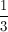Explanation:

For this problem we must understand Coulomb's Law. The force on charge A will be dircetly affected by the charge of Q1 and Q2. Q1 and Q2 are both negative, they will both attract charge A, and the net force will be reduced as they pull in opposite directions. Switching Q2 to a positive charge will result in a repulsive force on charge A which will be in the same direction as the attractive force between Q1 and A.

Example Question #1 : Electrostatics And Electrical Fields

Two point charges, Q1 and Q2, are placed on the x-axis. Q1 is placed at x = 0m and Q2 at x = 1m. If a positive charge, Q3, is placed on the x-axis at x = 0.25m, it experiences a net electric force of 0. Which of the following could be determined based on this observation?

Q1 and Q2 are both negative and have the same magnitude

Q1 and Q2 have the same sign, but Q1 has a smaller magnitude than Q2

Q1 and Q2 have opposite signs and the same magnitude

Q1 and Q2 have the same sign, but Q1 has a greater magnitude than Q2

Q1 and Q2 are both positive and have the same magnitude

Q1 and Q2 have the same sign, but Q1 has a smaller magnitude than Q2

Explanation:

In order for there to be 0 net force on Q3, the forces from Q1 and Q2 must be in opposite directions. So Q1 and Q2 must either be both attracting Q3 or both repelling Q3 (that is, Q1 and Q2 are either both negative or both positive).

Also, since these forces must have equal magnitude in order to cancel, such that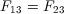, where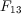is the magnitude of force between Q1 and Q3, and similarly for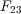. Recalling Coulomb’s law for electric force,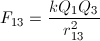and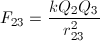(where Q1, Q2, and Q3 are the magnitudes of the respective charges). Since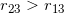, it must also be true that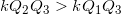for the two fractions to have the same value. So, Q2 must have a greater magnitude than Q1.

Example Question #4 : Electrostatics And Electrical Fields

Electronegativity is an important concept in physical chemistry, and often used to help quantify the dipole moment of polar compounds. Polar compounds are different from those compounds that are purely nonpolar or purely ionic. An example can be seen by contrasting sodium chloride, NaCl, with an organic molecule, R-C-OH. The former is purely ionic, and the latter is polar covalent.

When comparing more than one polar covalent molecule, we use the dipole moment value to help us determine relative strength of polarity. Dipole moment, however, is dependent on the electronegativity of the atoms making up the bond. Electronegativity is a property inherent to the atom in question, whereas dipole moment is a property of the bond between them.

For example, oxygen has an electronegativity of 3.44, and hydrogen of 2.20. In other words, oxygen more strongly attracts electrons when in a bond with hydrogen. This leads to the O-H bond having a dipole moment.

When all the dipole moments of polar bonds in a molecule are summed, the molecular dipole moment results, as per the following equation.

Dipole moment = charge * separation distance

Electronegativity is based on the principle that the closer an electron is to the atomic nucleus, where postive charge is concentrated, the more attractive force the positive charge can exert on the electron.

As a result, as atomic radius decreases by one half, what happens to the force pulling the electron toward that atom?

It is reduced by half

It goes up by a factor of eight

It stays constant

It doubles

Explanation:

This is a function of the equation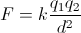.

In other words, as the distance between two charges doubles, the force between them goes increases, as the denominator decreases from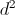to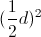.

Here, we are decreasing the distance by 1/2, decreasing the denominator by 1/4, so our force goes up by 4 times.

Example Question #1 : Electrostatics And Electrical Fields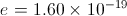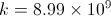How many electrons would it require to generate a 5 N attractive force on a +3 µC charge from 0.1 m away.

1.15 x 1013 electrons

2.3 x 10–13 electrons

0.68 x 1014 electrons

1.15 x 10–14 electrons

1.15 x 1013 electrons

Explanation:

To find the answer we must first solve for q, using Coulomb's Law. Then after finding q = 1.85 x 10–6 C, we must divide by 1.6 x 10–19 C to get the amount of electrons required to make such a charge.

Example Question #6 : Electrostatics And Electrical Fields

Batteries and AC current are often used to charge a capacitor. A common example of capacitor use is in computer hard drives, where capacitors are charged in a specific pattern to code information. A simplified circuit with capacitors can be seen below. The capacitance of C1 is 0.5 μF and the capacitances of C2 and C3 are 1 μF each. A 10 V battery with an internal resistance of 1 Ω supplies the circuit.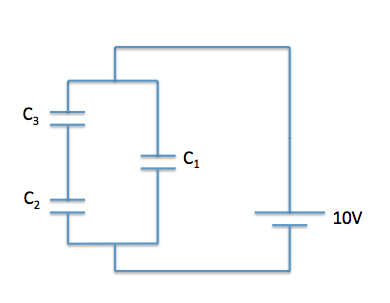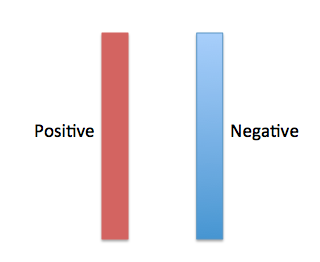Using the plate diagram above, what direction do the electric field lines point?

To the left

Into the page

Out of the page

To the right

To the right

Explanation:

As with a point charge, remember that electric field lines point from areas of high potential to areas of low potential. Areas of high potential have positive (+) charge and areas of low potential have negative (-) charge, thus the electric field lines point to the right.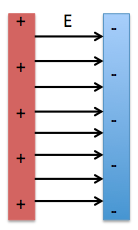Example Question #7 : Electrostatics And Electrical Fields

Which of the following equations best relates capacitance, charge, and distance between parallel plates as they pertain to the electric field,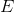?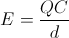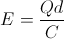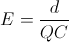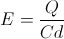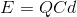Explanation:

This question forces us to combine two equations.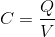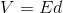Using substitution, we can solve for the electric field with the variables given in the question stem.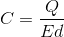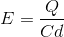Example Question #8 : Electrostatics And Electrical Fields

Batteries and AC current are often used to charge a capacitor. A common example of capacitor use is in computer hard drives, where capacitors are charged in a specific pattern to code information. A simplified circuit with capacitors can be seen below. The capacitance of C1 is 0.5 μF and the capacitances of C2 and C3 are 1 μF each. A 10 V battery with an internal resistance of 1 Ω supplies the circuit.Using the plate diagram above, in what direction do the electric force lines point?

Into the page

To the left

Out of the page

To the right

To the right

Explanation:

As with a point charge, remember that electric force lines point away from positive charge and towards negative charge. As can be seen in the image, electric force lines point to the right. As an aside, electric field lines point in the same direction as electric force lines, so if you know the direction of one, you also know the direction of the other.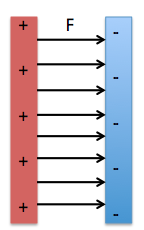Example Question #9 : Electrostatics And Electrical Fields

Consider a spherical shell of radiushas a charge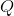.

What is the electric field at a distance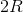from the surface of the shell?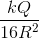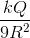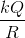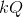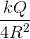Explanation:

According to the shell theorem, a charged spherical shell can be treated as a point charge at the center of the spherical shell. The equation for the electric field of a point charge is: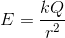In this case,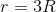since we are at a distance offrom the surface of the shell and therefore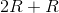away from the shell's center. Plug into the equation for electric field.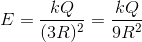Example Question #10 : Electrostatics And Electrical Fields

Consider a spherical shell of radiushas a charge.

What is the electric field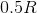away from the center of the shell?